International
Tables for
Crystallography
Volume D
Physical properties of crystals
Edited by A. Authier

International Tables for Crystallography (2013). Vol. D, ch. 1.11, p. 281

## Section 1.11.7. Glossary

V. E. Dmitrienko,a* A. Kirfelb and E. N. Ovchinnikovac

aA. V. Shubnikov Institute of Crystallography, Leninsky pr. 59, Moscow 119333, Russia,bSteinmann Institut der Universität Bonn, Poppelsdorfer Schloss, Bonn, D-53115, Germany, and cFaculty of Physics, M. V. Lomonosov Moscow State University, Leninskie Gory, Moscow 119991, Russia
Correspondence e-mail:  dmitrien@crys.ras.ru

### 1.11.7. Glossary

| top | pdf |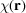local susceptibility tensor in direct space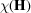Fourier components of the local susceptibility tensorreciprocal-lattice vectorpolarization vector of an X-ray wavewavevector of an X-ray wave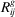matrix corresponding to point-group operatorpolarization vector perpendicular to the scattering planepolarization vector in the scattering planeBragg angleazimuthal angle of rotation about a reciprocal-lattice vectorvector potential of the electromagnetic wavemomentum of an electronfrequency of an electromagnetic wavewavelength of the radiationenergy of a discrete atomic levelspin of an electron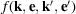scattering amplitudescattering vector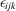Levi-Civita symboltransition frequency for states a and cenergy width of the excited state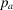probability that the state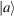of the scatterer is occupiedtensor atomic factor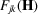structure-factor tensor of rank 2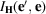intensity of the reflection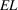notation of the electric multipole transition.: the dipole;: the quadrupole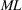notation of the magnetic multipole transition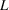orbital moment of electron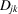dipole–dipole tensor atomic factorsymmetric part of the dipole–dipole tensor atomic factorantisymmetric part of the dipole–dipole tensor atomic factorthird-rank tensor describing the dipole–quadrupole resonant X-ray scattering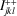part of the third-rank tensor invariant under time inversion and symmetric under the permutation of j and k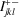part of the third-rank tensor non-invariant under time inversion and symmetric under the permutation of j and k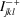part of the third-rank tensor invariant under time inversion and antisymmetric under the permutation of j and k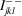part of the third-rank tensor non-invariant under time inversion and antisymmetric under the permutation of j and kfourth-rank tensor describing the quadrupole–quadrupole resonant X-ray scattering Tr trace of matrixmagnetic moment of an atom YLM spherical tensorcomponent of the spherical tensor depending only on the incident and scattered radiationcomponent of the spherical tensor associated with the tensor properties of the absorbing atom

### References

Di Matteo, S., Joly, Y. & Natoli, C. R. (2005). Detection of electromagnetic multipoles by X-ray spectroscopies. Phys. Rev. B, 72, 144406.
Paolasini, L. (2012). Magnetism in condensed matter: resonant and magnetic scattering by synchrotron radiation. In Italian School on Magnetism, Pavia 2012, pp. 1–83. Universita di Pavia.